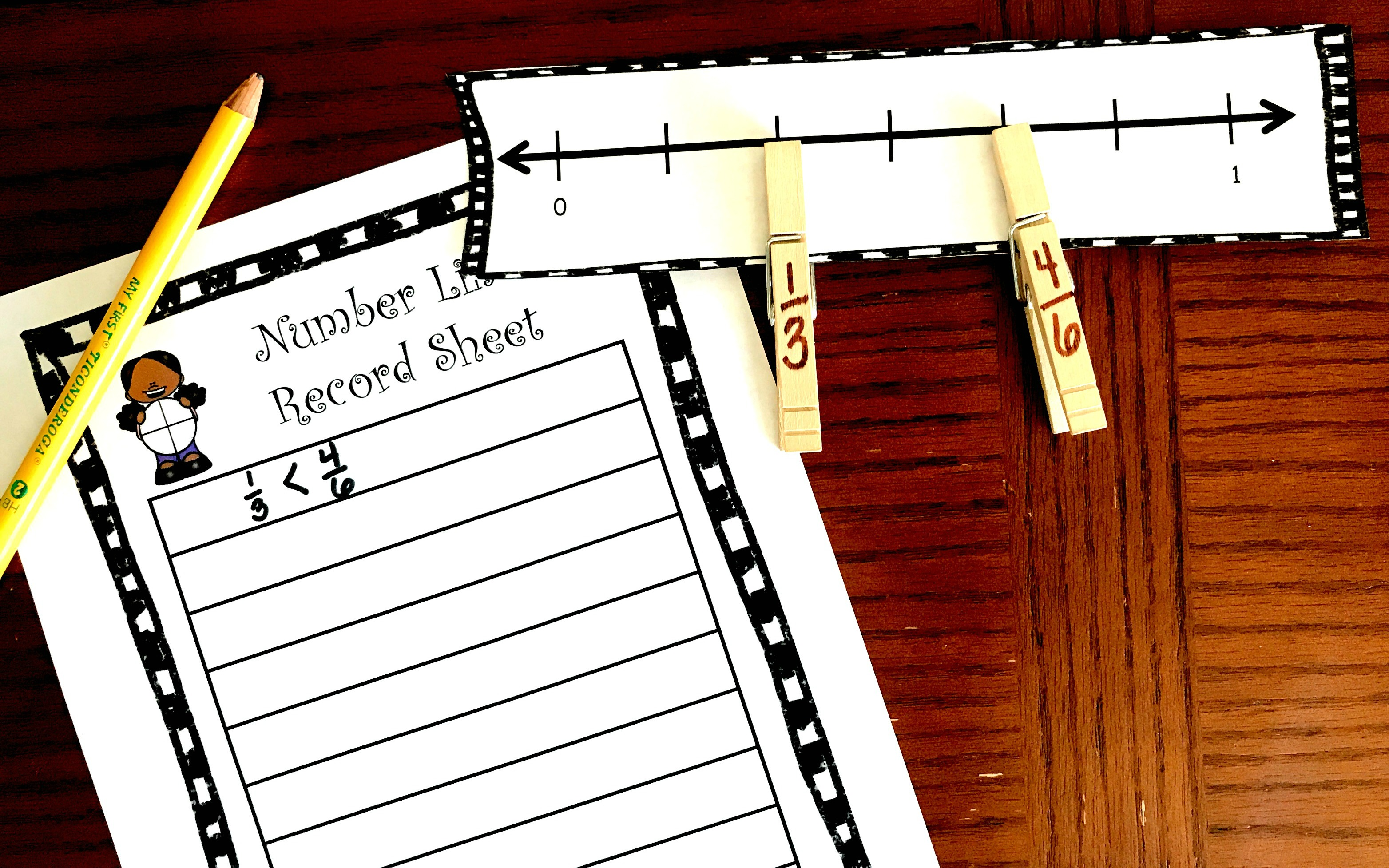# FREE Printable Hands-On Comparing Fractions Activity Using A Number Line

5 Shares

This FREE hands-on comparing fractions activity has children placing fractions on a number line using clothes pins to help them compare and order fractions.

Comparing fractions can be a tricky concept, but hands-on practice is a wonderful way to build fractions sense and help children visually see how fraction compare.

One way I love to teach comparing fractions is by using a number line. Creating number lines and placing fractions on them solidifies their understandings of numerators, denominators, equivalent fractions, and comparing fractions.

This hands-on comparing fractions activity builds on a child’s understanding of number lines and fractions. If you haven’t played with fractions and number lines yet, you may want to do some journal work and then move to this activity.

These free task cards are a great place to start, as they will help children create number lines and place fractions on them.

## Prep – Work

This is a pretty simple activity, and the only part that requires some time is writing the fractions on the number line.

1. First, print off the number lines on card stock paper and cut out.
2. Next, it is time to write down some fractions.

– Write ½ and then all the fractions for fourths and eights for the first number line.
– The next number line is all about thirds and sixths so you will write 1/6, 2/6, etc for this number line.
– Number line three focuses on fifths and tenths, so the pins fo this line will be 1/5, 2/5, 1/10, 2/10 etc.

3. Finally, print off the record sheet and place in a sheet protector if you want to reuse it. Or you could have the students turn it in for a grade.

## How to Use Hands-on Comparing Fractions Activity

The directions are very straightforward. The students draw two clothespins from a bag and then place them on the number line. Now they can easily compare the two fractions, and record their findings on the recording sheet.

Once they have written down their answer, they put the two clothes pins back in the bag and draw two more.

They do this until they have filled their record sheet.

#### Example 1:

For the first number line, you can see that there are eight spaces between the zero and the one. This means that each line represents 1/8.

Let’s say that the student drew 3/4 and 7/8. Well, the 7/8 is easy. They simply count over seven lines and place the clothespin there. But the 3/4 requires a little equivalent fraction knowledge.

• They could do the equivalent fraction formula and figure out the 3/4 is equal to 6/8.
• Or they could take the number line, and divide it into four equal parts to see where 3/4 falls.

Once they do that they can place the 3/4 clip on the correct line, and now it is easy to see that 7/8 is greater than 3/4.

#### Example 2:

For the last number line, things get a little more complicated. There is only a 1/2 mark on the number line and all the clothespins have been thrown into the bag.

When the student draws the pins, they may get lucky and get an easy one like 1/3 and 3/5. Most children will be able to recognize that 1 out of 3 is less than 1/2, while 3 out of five is greater than 1/2. So the 1/3 clip goes to the left of one-half and the 3/5 goes to the right of 1/2. Therefore, 1/3 is less than 3/5.

But things could get a little more complicated if they drew 1/3 and 2/5. We know they are both less than 1/2, so a little legwork must be done. We could always find the common denominators, and then divide the line into fifteen parts. At this point, it is easy to place 1/3 or 5/15 and 2/5 or 6/15 on the number line.

Or we could use the other two number lines, line them up exactly, and then place the fractions on them. You can see that 2/5 is greater than 1/3!!

I hope this hands-on comparing fractions activity continues to build fraction sense with your children.

You’ve Got This

Rachel

Comparing-and-Ordering-Fractions

You may also like:

This free comparing fractions game. It is a great way to review this skill.

Or get all my comparing fractions activities in one easy download.5 Shares